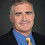## Thursday, March 16, 2023

### Identities in Computational Complexity

Guest post by Josh Grochow

On the birdsite, Jay Cummings tweeted:

And it got me thinking about identities in computational complexity. At first I thought: how many could there be? 10? After some thought and with some help, there turn out to be quite a few more than that, and I think they make a pretty good list!

• Some are relatively simple alternative characterizations, such as the various definitions of PH; most are pretty nontrivial theorems.
• I didn't include existentially quantified oracle results (such as "there exists A such that PA=NPA"), despite them being some of my favorite results, because there'd be waaaay too many of those. Probably thousands? Ask Lance. In some circles he's known as the "oracle oracle" - as in, he's who you go ask if you want to know if a certain oracle exists. I did include identities involving oracles where one side of the identity didn't have an oracle, such as EXP=NPRKt or AlmostP=BPP (AlmostP is the class of languages L such that {A : L is in PA} has measure 1).
• I also didn't include some important conditional equalities, such as "EXP in P/poly iff EXP=MA" or "NP in BPP iff NP=RP". I guess it's not really an "identity" if it's conditional. Games are only fun if the rules are at least a little constraining!
• There are some surprising containments that either aren't equalities, or aren't known to be equalities, and I didn't include those either, despite some of them being very important. For example, BPP in Σ2P, other depth reduction results (such as the chasms at depth 4 and 3), and Beigel-Tarui/Yao.

One could teach a class based on a list like this and cover a lot of good ground, but I think it'd be sad to leave out any lower bounds. Actually, by my estimate, if you were teaching from "scratch" (say, starting after an undergrad algorithms class), this list, and all its implied prerequisite material, is already probably the equivalent of about 3-4 semester-long classes!

What other complexity identities did I miss?

Finally, without further ado, a list of (some) identities in computational complexity:

Space classes

DET=LGapL (see Update 1)

Interactive Proofs

NP=PCP(O(log n), 3)

IP=PSPACE

AM = MAM = AMAM = ... [From Albert Atserias @atserias]

Alternating Turing machines

Σk P = ∃ Pik-1 P = NPΣk-1 P = ΣkTIME(poly(n)) [from Lance]

Circuit classes within P

SAC1 = LogCFL [from Michael Levet @Michael_Levet]

REG = DSPACE(O(1)) = NSPACE(O(1)) [from Levet]

REG=DTIME1tape(o(n log n))

Quantum

QMAlog (1)=BQP [from Martin Schwarz @martin_schwarz]

QMAlog (poly)=QCMA [ditto]

QAC0f = QNC0f [from Ale `Scinawa' Luongo @scinawa]

QMA(k) = QMA(2) for any k ≥ 2 [from Sarvagya Upadhyay @sarvagya82]

Algebraic complexity

For bilinear maps, tensor rank=Theta(algebraic circuit size)

Logical characterizations

FO=AC0

AC=NC=FO[poly(log)]

P = FO + LFP on ordered structures [thanks to Lance, and Michael Levet]

Kolmogorov-random strings as oracles

Almost-classes

1. March 17th update, h/t Eric Allender: Using Cook's original definition of DET as problems NC1-reducible to the integer determinant, apparently this equality is not known! See Eric's recent guest column in SIGACT News for details and a \$1000-prize related to this question, along with many other interesting open questions.

1.Not exactly a complexity identity, but also "Turing equivalence" leads to some suprising "identities", too.

2.Nice Thursday complexity post.

3.Many interesting ones in regular languages too: MSO=REG, FO=Star-Free, ...

4.To explain upon the "AC0 = FO":

If we extend FO with arithmetic predicates (i.e., addition and multiplication), denoted FO(+,x), then we get that FO(+,x) = DLOGTIME = DLOGTIME-uniform AC0.

If we have FO with the ability to use an arbitrary numerical predicate, denoted FO(Arb), then we get that FO(Arb) = non-uniform AC0.

Then, in line with the above anonymous comment, if we extend FO with an ordering relation, denoted FO(<) (i.e., FO over ordered structures), then we get that FO(<) = star-free regular languages.

Subtle distinctions but important because FO(<) \subset FO(+,x) \subset FO(Arb).

5.Do you mean MIP*=RE?

- Michael C.

1.RE (recursively enumerable) and CE (computably enumerable) are the same class. I find the latter more appealing.